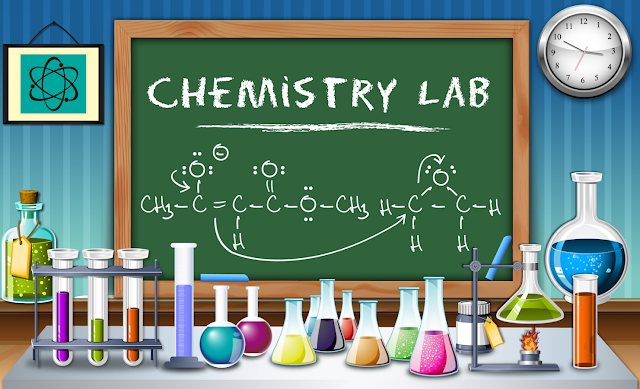Thursday, 4 August 2016

Chemistry MCQs practice PIEAS entry test taken from past papers

Chemistry

1) Which is a exothermic process?
(a) melting
(b)freezing
(c)evaporation
(d)condensation
2) Which force between molecules is the strongest force
A) Ne
B) CH4
C)CO2
D) H2O
3) What is the symbol of tungsten. .
A)Te
B) W
C) Ti
4) What is the morality of water..
A) 1 molar
B) 55.5 molar
C) 5.5 molar
D) 550 molar
5) What is hybridization of orbitals of Ammonia NH3...??
A)sp
B)sp2
C)sp3
D) dsp3
6) Oxidation state of hydrogen in metal hydrides is
(a) +1
(b) +2
(c) -1
(d) -2
7) Which of the following is least related to polarity
(a)Normal boiling point
(b)Molar mass
(c)Solubility in water
(d)Critical Temperature
8) rate equation wly 2 3 ques
9) In aqua regia the ratios of HCL and HNO3 is
(a) 2:3
(b) 3:1
(c) 1:3
(d) 1:2
10) Most stable form of oxygen is
(a) O^2-
(b) O^2+
(c) O^1-
(d) O^1+
Also read:Mathematics MCQs PIEAS from entry test
11)If a sample of nitrogen is inserted in a needle and placed in a beaker. If its volume is increased to three times and the absolute temperature is increased 2.8 times then the pressure on it will be
(a) 0.8P
(b) 4.5P
(c) 1.3P
(d) None of these
12) Which of the following will be most dissolved in water
(a) Polar
(b) Non polar
(c) Neon Gasses
(d) Gasses like O2 and H2
13) Which is the oxidizing agent in the following reaction
H^2+ +~~Mn^2+ +~~Fe^2+===>~~H2O +~~Fe^3+~~ ~~+MnO^2+~~
(a) H2O
(b) Fe^2+
(c) H^2+
(d) Mn^2+
14) Which of the following has different mass from the other three given options?
(a) 3 ethyl pentane
(b) 2,2 dimethyl butane
(c) 2 methyl butane
(d) 2,3 di ethtl pentane
15)  Order of reaction of Rate=[B]^2[A]^1[C]^2
(a) 1
(b) 3
(c) 4
(d) 5
16) Mass percent of NaCl when 20g of it is dissolved in 100g of water is
(a) 20
(b) 30
(c) 58.5
(d) 14
(e) 5The following MCQs came in PIEAS test but the option are not in mind so try to solve them directly.
1)Which of the following is not a pair of Lewis acid and Lewis base?
2)Which of the following contains the maximum number of oxygen atoms?
3)Which of the following best defines covalent bond?
4)Balance the following reaction and tell the number with HCLO4?
5)Which of the following is exothermic?
Which if the following is most dissolved in water?
6)What is the Kc value of the following reaction?
7)Which of the following is most stable?
8)Which symbol represents tungsten?
9)What is the order of reaction of the following?
10)What is the ratio of mole fraction of glucose if 180g of it is dissolved in 100g water?
11)Nitrogen is filled in a needle and placed in a jar. Volume of it is increased to three times and absolute temperature is increased 2.8 times. What is the pressure?
12) Which of the following is the oxidizing agent in the given reaction?
13) Hybridization in NH3?
14) Which of the following best describes the large number of compound forming of carbon?
(Please provide answers in comments and share it with those who will also give PIEAS test).